Next: Passive Element Lines Up: Device Models Previous: Default Models   Contents   Index

## Analysis at Different Temperatures

All input data for WRspice is assumed to have been measured at a nominal temperature of 25C, which can be changed by use of the tnom parameter on the .options control line. Note that this is the same default temperature used in HSPICE, but is not the same as in Berkeley SPICE3 or in WRspice releases prior to 3.2.15, which was 27C.

This value can further be overridden for any device which models temperature effects by specifying the tnom parameter on the model itself. The circuit simulation is performed at a temperature of 25C unless overridden by a temp parameter on the .options control line. Individual device instances may further override the circuit temperature through the specification of a temp parameter on the instance.

Temperature dependent support is provided for resistors and semiconductor devices. The details of the temperature adjustments can be found in the description of the models. For details of the BSIM temperature adjustment, see  (BSIM1),  (BSIM2), and  (BSIM3).

Temperature appears explicitly in the exponential terms of the BJT and diode model equations. In addition, saturation currents have a built-in temperature dependence. The temperature dependence of the saturation current in the BJT models is determined by:

Is(T1) = Is(T0)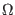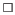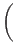exp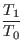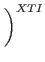where k is Boltzmann's constant, q is the electronic charge, Eg is the energy gap which is a model parameter, and XTI is the saturation current temperature exponent (also a model parameter, and usually equal to 3).

The temperature dependence of forward and reverse beta is according to the formula: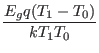(T1) =(T0)where T1 and T0 are in Kelvin, and XTB is a user-supplied model parameter. Temperature effects on beta are carried out by appropriate adjustment to the values of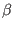, ISE ,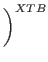, and ISC (WRspice model parameters bf, ise, br, and isc, respectively.

Temperature dependence of the saturation current in the junction diode model is determined by:

IS(T1) = IS(T0)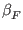exp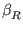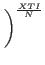where N is the emission coefficient, which is a model parameter, and the other symbols have the same meaning as above. Note that for Schottky barrier diodes, the value of the saturation current temperature exponent, XTI , is usually 2.

Temperature appears explicitly in the value of junction potential,(in WRspice, phi), for all device models. The temperature dependence is determined by: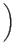(T) =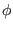ln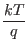where k is Boltzmann's constant, q is the electronic charge, Na is the acceptor impurity density, Nd is the donor impurity density, and Ni is the intrinsic carrier concentration.

Temperature appears explicitly in the value of surface mobility,(or u0, for the MOSFET models. This temperature dependence is determined by:(T) =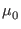The effects of temperature on resistors is modeled by the formula:

R(T) = R(T0)[1 + TC1(T - T0) + TC2(T - T0)2]

where T is the circuit temperature, T0 is the nominal temperature, and TC1 and TC2 are the first and second-order temperature coefficients.Next: Passive Element Lines Up: Device Models Previous: Default Models   Contents   Index
Stephen R. Whiteley 2020-07-23